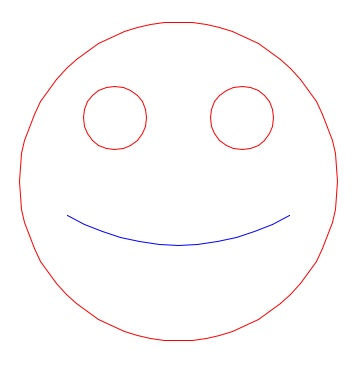# A bit more on graphing under software control

Up a level : Nspire and Lua
Previous page : Nspire and 3D plotting before it was a part of the software
Next page : A favourite tool - the LocusHave it under control

In the previous page I used the spreadsheet to control the graphs, but one can do that under program control too.  As with the spreadsheet one need to create a graph window, and some vectors of points. Then one make scatter plots of the data, and set all the desired properties of the plots in the graph window. One can then add points to the vectors, remove points and move points using programs.

Example code:

```Define smily(x,y)=
Prgm
Local i
i:=1
For t,0,360,5
xx[i]:=x+5*sin(t°)
yy[i]:=y+5*cos(t°)
i:=i+1
EndFor
xx[i]:="_"
yy[i]:="_"
i:=i+1
For t,0,360,15
xx[i]:=x+1*sin(t°)+2
yy[i]:=y+1*cos(t°)+2
i:=i+1
EndFor
xx[i]:="_"
yy[i]:="_"
i:=i+1
For t,0,360,15
xx[i]:=x+1*sin(t°)-2
yy[i]:=y+1*cos(t°)+2
i:=i+1
EndFor
i:=1
For t,180-30,180+30,5
xm[i]:=x+7*sin(t°)
ym[i]:=y+7*cos(t°)+5
i:=i+1
EndFor
EndPrgm```

(Observe that the above code cannot be copy-pased onto an Nspire document, it is just for show.  I had to change a few characters to make the list readable.-)

Running smily(0,0) would (after setting the right colours, an so on) give us:One can then move the figure around by calling smily(x,y) for different values of x and y.  To make it a bit more interesting I added two sliders, one for x and one for y, and to make the call of the smily function to  happen every time one has changed either x or y, I added a call to mily(x,y) in a notebook window in the same document.

The document: smily.tns.

The above could have been done in easier ways, but this was basically done just to illustrate the principle.

A short summary

• One can emulate plot commands using scatter plots
• One can make it event driven by running calls to programs in a notebook page. Those programs will run every time one of the variables in the function call changes.
• One can also get a similar effect by using tables in a spreadsheet page. Changes in any variables used in the spreadsheet will cause the cells to be recalculated, and if the cells are used as coordinates for points in a graph, the the graph will update too.
• If one select that the points in the graph are connected, one can still make several disjoint parts of the graph by separating the values in the tables by something that is not a number, e.g. “_”.

A note about another trick

In Nspire, see the the degree sign as a function that turns the angle in degree to an angle in whatever angle mode you happen to have. Use this on angle values (that are in degrees) – this will make your program angle mode independent.Up a level : Nspire and Lua
Previous page : Nspire and 3D plotting before it was a part of the software
Next page : A favourite tool - the LocusLast modified: Feb 17, 2017 @ 21:33